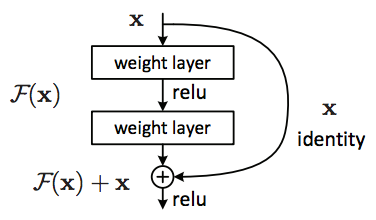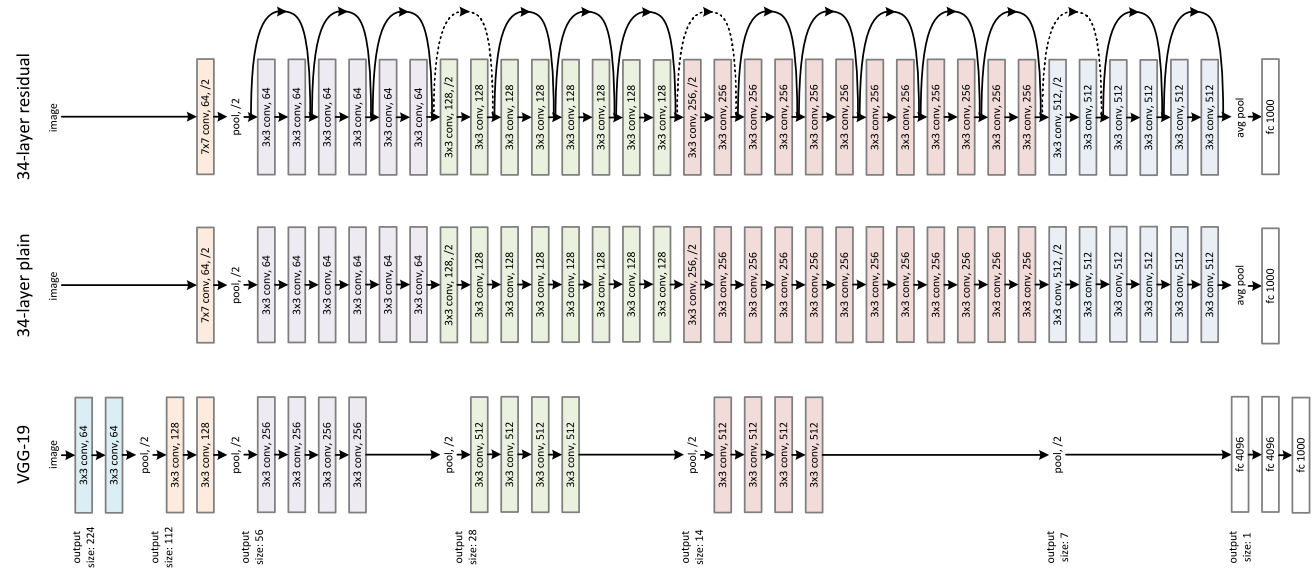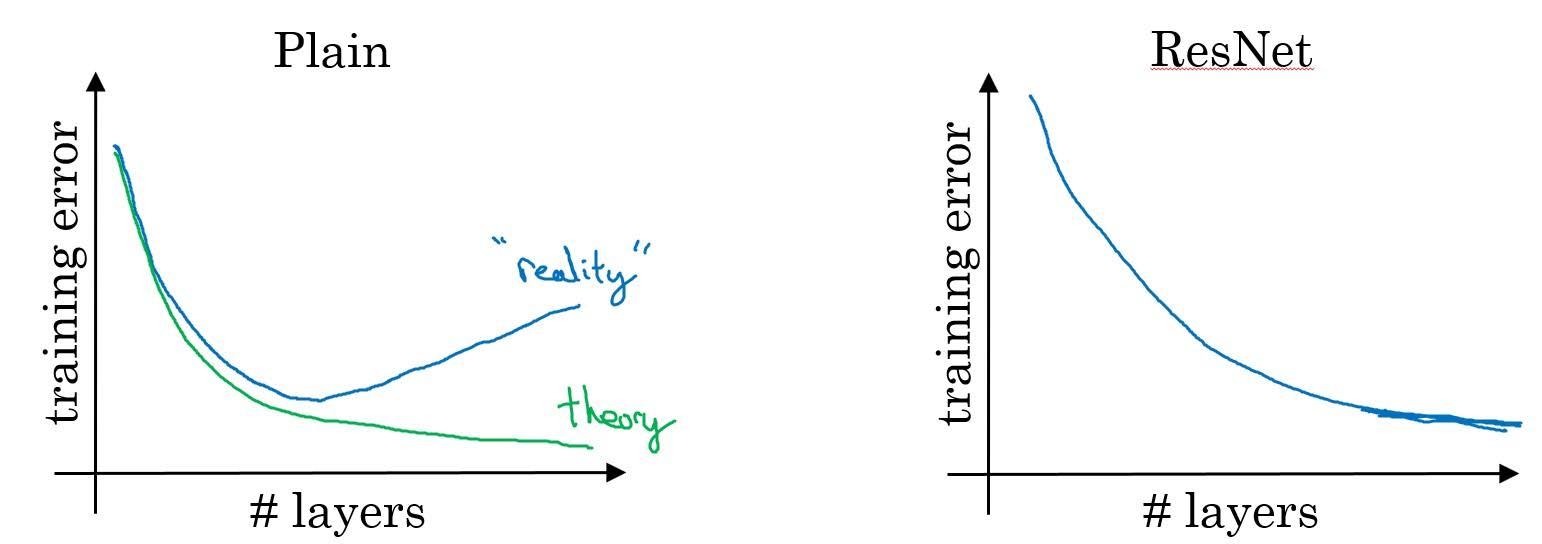# ResNet

Categories:

Updated:

He et al., 2015. Deep residual networks for image recognition

# ArchitectureResNet is composed of many residual blocks that look like above.

The first network in the below image is an example of ResNet.# Why ResNet?

When plain neural network becomes too deep, its error increases in reality, unlike in theory.ResNets perform well with deep networks. But why?

It’s because identity function is easy for residual blocks to learn.

When $W$ and $b$ is close to 0, $a^{[l+2]}=a^{[l]}$.

So adding residual block to somewhere in a neural network at least doesn’t hurt its performance but only can improve performance.

# Keeping Dimensionalities

To accomplish $a^{[l+2]} = g(W^{[l+1]}a^{[l+1]} + b^{[l+1]} + a^{[l]})$, dimensionalities of $a^{[l]}$ and $a^{[l+2]}$ should be same.

So we mostly use same convolutions. If the dimensionalities don’t match, we multiply $W_s$ to $a^{[l]}$. $a^{[l+2]} = g(W^{[l+1]}a^{[l+1]} + b^{[l+1]} + W_sa^{[l]})$ For example when shape of $a^{[l+2]}$ is 256 and shape of $a^{[l]}$ is 128, $W_s$ should be a weight matrix of 256x128.

Categories: